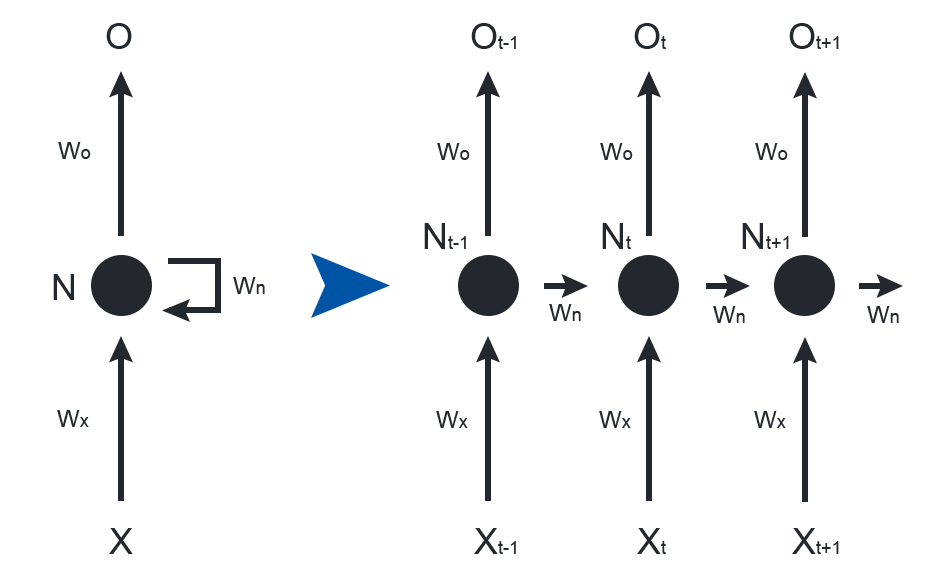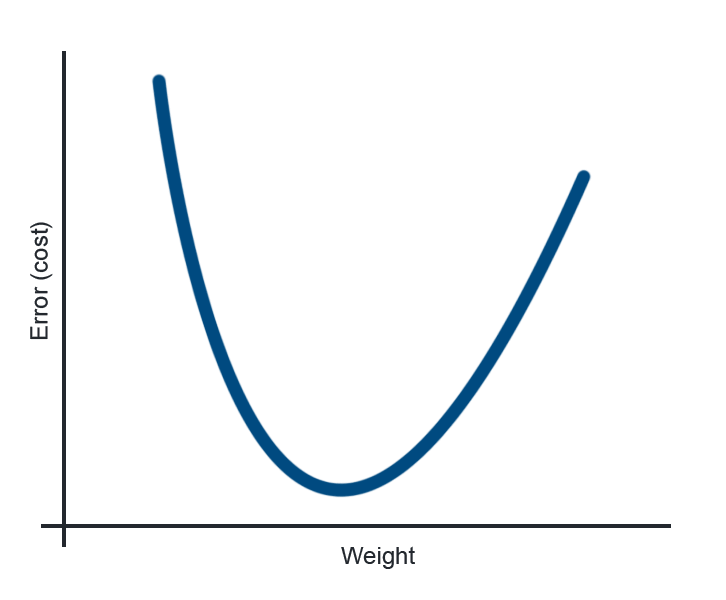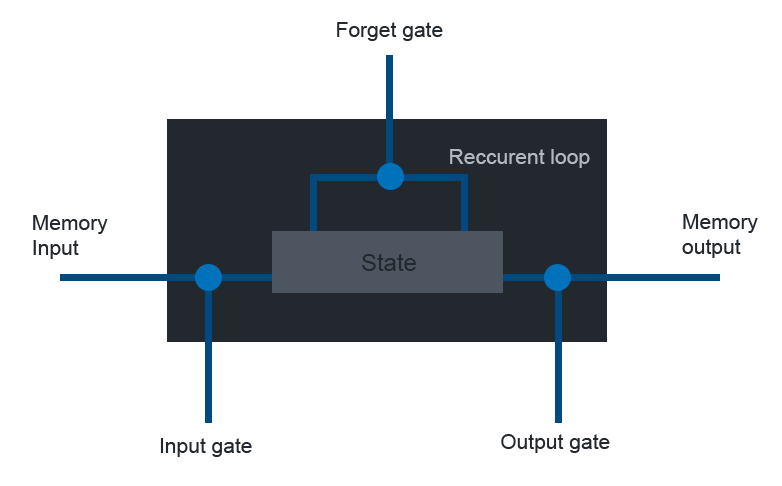# Neural Networks

2 March 2018 | Peter Swift | About a 4 minute read

### Introduction

Neural networks are a fantastic tool for understanding and extracting information from datasets, especially as the data becomes too large and complex to be understood by static analysis tools. Without an understanding for which network is appropriate for a given data set, the network may fail and miss important trends and connections within the data.

The purpose of this blog is to give a brief look into a type of network designed to capture sequential dependencies and is intended for someone familiar with the basic concepts of neural networks.

### RNN

Recurrent neural networks (RNNs) link outputs back into the nodes that produce them (or nodes in the same layer), forming a recurrence. This simulates a state in time that can then inform the next state so as to accurately model sequentially dependent events (data that depends on previous information). Regular feed-forward (one-way) networks inherently treat the data as independent even though it may not be, such as words in a sentence.

This kind of RNN can be rolled out into one without loops by treating each layer as a state in time. This network now obviously features many hidden layers, showing itself to be a deep learning technique.Two representations of a recurrent neural network – on the left, the more concise recurring loop, and on the right the loop is ‘rolled out’ to show the node in different states in time. Note that there is an output for each state, and that the weights do not change with time.

Unfortunately, training deep neural networks becomes harder the more layers they contain. The standard back-propagation algorithm uses a concept known as gradient descent to minimise the error – the error (or cost) can be modelled as a mostly convex function of the weights, as there must be some optimal combination of weights with the minimum error. This minimum can be found by repeatedly moving in the direction that has the largest negative gradient or steepest line, in an attempt to find the optimum weights. Mathematically, this method requires repeated use of the chain rule, which can lead to early layers learning much slower than later ones (the vanishing gradient problem) or vice versa (exploding gradient). If the multiplier is small (less than one), then constantly multiplying by this value can cause the gradient to vanish, while large values can lead to the gradient exploding in much the same way. The more multiplications, the more acute the problem, and as early layers require the most multiplications, it is these layers that are most affected.A visual representation of the cost as a function of weight (one dimension for clarity) – the minimum error can hence be found by moving in the direction of the largest negative gradient from a randomly initialised weight.

### LSTM

To fix the issues that occur over long times, it is possible to replace standard neurons with long-short term memory (LSTM) cells. Each LSTM cell is a combination of logical gates designed to store old values for arbitrary lengths of time, avoiding the exponential multiplication that can occur in a normal RNN. This has the practical intuitive effect of being able to use information from an arbitrarily long time ago with as much importance as something that occurred more recently, or in other words, long term memory.A high-level diagram of a LSTM cell – the memory cell input comes from previous cells, and the memory cell output goes to future cells. The input and output gates determine whether these values affect the state of the cell, and the forget gate controls the memory of the previous state.

### Conclusion

Hopefully, this high level overview has given some insight into the inner workings of RNNs, and therefore highlighted the need to understand which neural network would be appropriate for a set task. If anyone reading this blog post would like some more information about these particular networks or just neural networks in general then feel free to contact me at my email address [email protected]